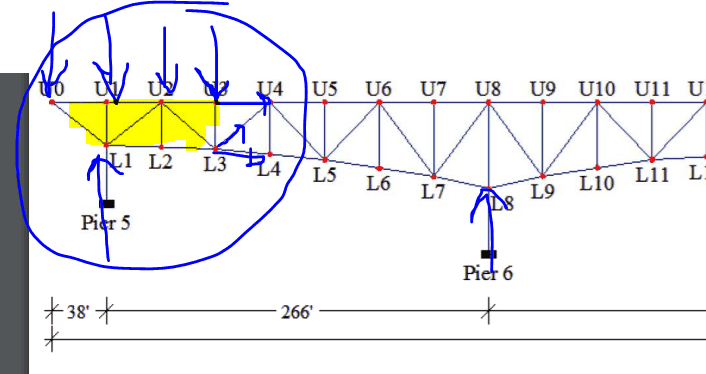# Calculate Force Member in Truss

• lc99
lc99

## Homework Statement## The Attempt at a Solution

I just want to know if I am doing this right... So, my homework is to calculate the members of a truss. So, here i have a picture of a bridge (truss). I am suppose to calculate the member going from U3 to U4. Thus, I am going to cut the section off (the yellow highlighted part) and do a summation of moments about L3, so that i can solve for the member U3-U4.

Is this correct? Since with method of sections, i can just ignore the rest of the bridge and focus on the left side?

Also, is the member U6-L6 (vertical pillar) a zero force member? So that the member's force =0?

Homework Helper
Gold Member

## Homework Statement

View attachment 223509

## The Attempt at a Solution

I just want to know if I am doing this right... So, my homework is to calculate the members of a truss. So, here i have a picture of a bridge (truss). I am suppose to calculate the member going from U3 to U4. Thus, I am going to cut the section off (the yellow highlighted part) and do a summation of moments about L3, so that i can solve for the member U3-U4.

Is this correct? Since with method of sections, i can just ignore the rest of the bridge and focus on the left side?

Also, is the member U6-L6 (vertical pillar) a zero force member? So that the member's force =0?
Yes your method of sections is correct . I assume you would already know all the applied loads and reaction load at pier 5.
Member U6L6 is not a zero force member because although no load Is applied to it, members L5L6 and L6L7 are not colinear, since the bottom chord is curved and not a straight line. Those members have to lie along a straight line in order for the vertical member to have no force in it.

•CivilSigma
lc99
Yes your method of sections is correct . I assume you would already know all the applied loads and reaction load at pier 5.
Member U6L6 is not a zero force member because although no load Is applied to it, members L5L6 and L6L7 are not colinear, since the bottom chord is curved and not a straight line. Those members have to lie along a straight line in order for the vertical member to have no force in it.

thanks. i kinda doubt that it was a zero force member. I kinda didnt want to go through finding the joints around the vertical force member.